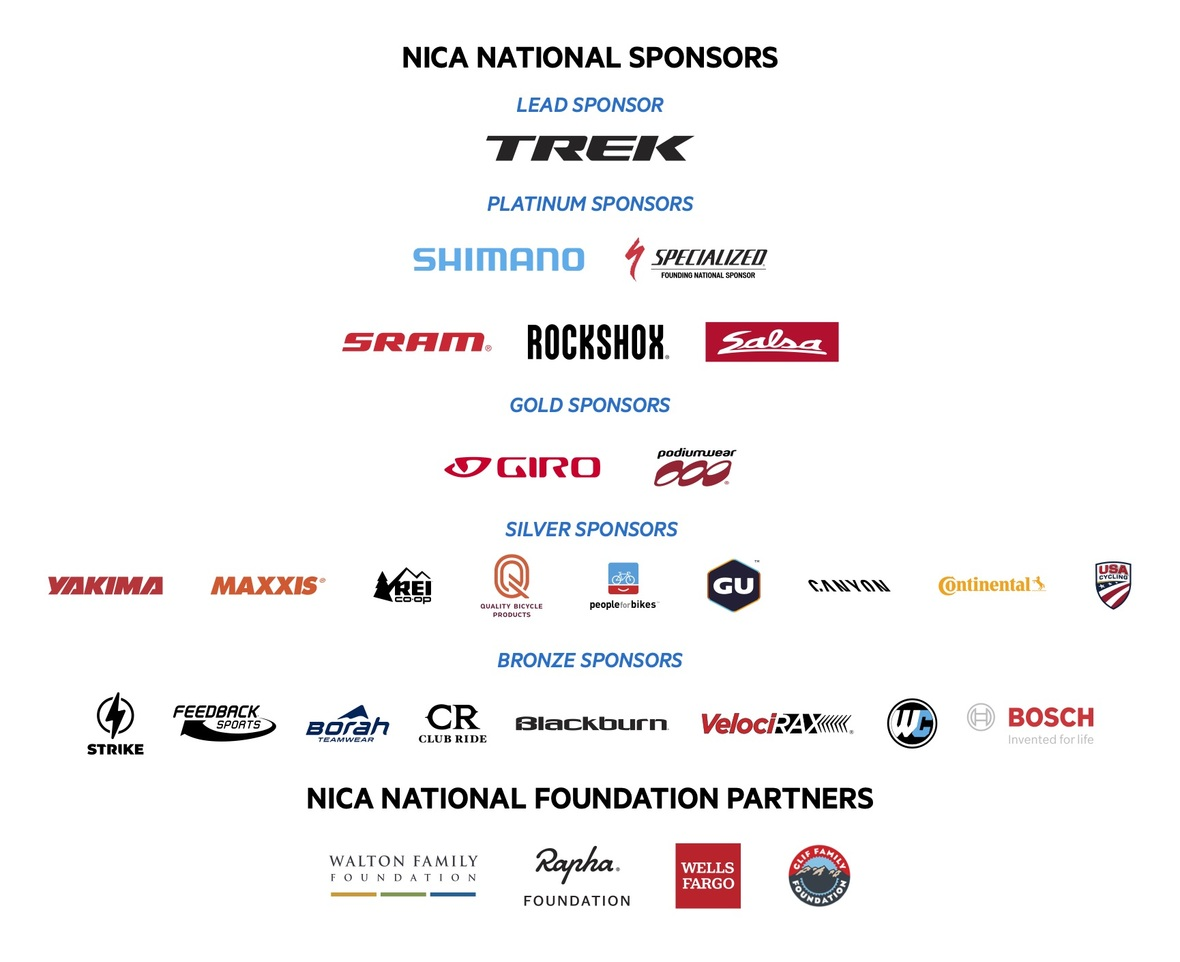Like   Tweet   Pin   +1   in
 /* styles */ Hello you amazing coaches, Racing has begun across the country in our spring leagues! Texas, Florida, Alabama, and Southern Nevada all held races the weekend of March 6th and 7th! Ohio held its inaugural leaders summit (virtually) and Indiana was able to hold their leaders summit this last weekend! Fall league coaches have started registering for their 2021 season. While coaches prepare for another unprecedented year I see a lot of hope and excitement. Mountain biking is awesome! As you plan your seasons, take time to celebrate what makes our sport awesome. Celebrate challenge by choice and individual goal setting. Craft season and session plans that allow every student-athlete and coach to achieve their goals. Win the race to the right finish line, by building strong minds, bodies, character, and community through cycling. Coaches matter! Thanks for being a coach, Mike McGarry and the Coach Licensing Team In This Issues -Game of the month -2020 NICA Awards Recipients Announced -Social Media Best Practices -How to Find Your Coach Credentials -Trek Pathfinder Scholarship -The Sparkle On Scholarship -Campfire Chat -Coach Licensing Updates for Fall Leagues -Giddy Up for Good Challenge -Strawberry Meet Hibiscus GU -Pearl Izumi - Temporary Coach Benefit!
 table div table+table+table div table{width:100%;padding:0}table div table+table+table div table img{width:96.23%;padding:0;float:none}table div table+table+table div table td{width:100%;padding:0 1.88% 18px}/* styles */## Slalom Relays

Where: Open Space
Objective: I can build a positive team culture. I can have fun. I can navigate a slalom course forwards and backwards
101 Skills: Bike-Body Separation, Braking, Neutral/Ready Position
Setup: Divide your group into equal teams. Build a side by side dual/multi slalom course using soccer cones or flags. Student-athletes all start at the same end.
Rules: Student-athletes race forwards through the slalom course. Once they reach the end of the course, they dismount and push the bike backwards while holding onto their handlebars and slaloming through the cones.
Video: If you are having trouble visualizing this here is a video of the LaCrosse Area Mountain Bike Team doing the Slalom Relay at the end of their first week of practice from 2019.
Reflection Question: What could make this game more fun/challenging?

What is your favorite game to play at practice? Use this NICA Game Submission Form to share your favorite games.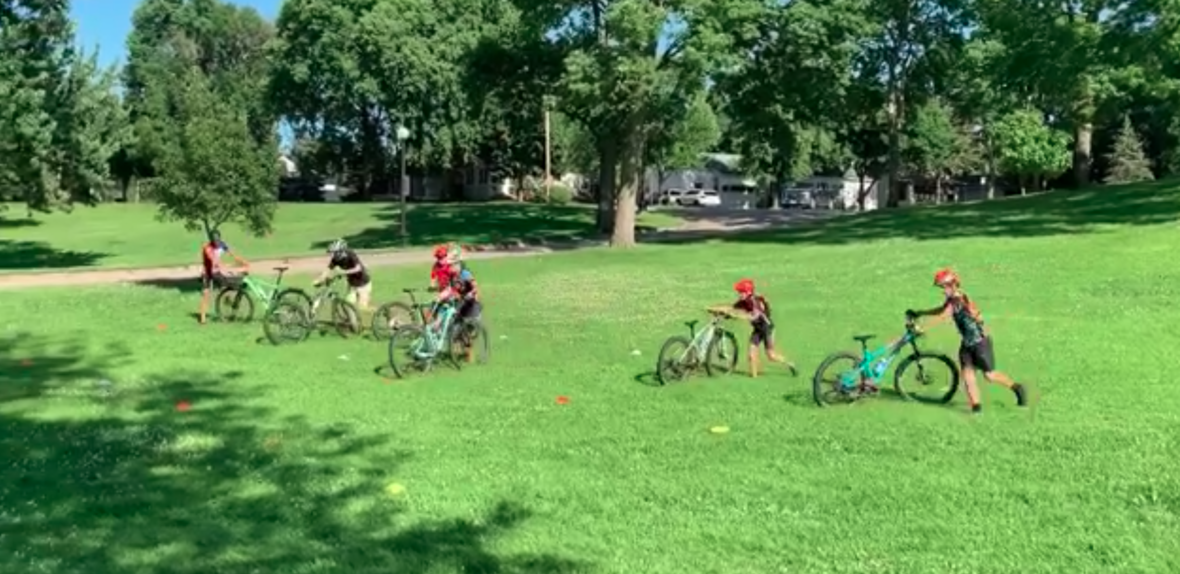LaCrosse Area Mountain Bike Team competing in a slalom relay! Wisconsin League
 table div table+table+table+table+table+table+table div table{width:100%;padding:0}table div table+table+table+table+table+table+table div table img{width:96.23%;padding:0;float:none}table div table+table+table+table+table+table+table div table td{width:100%;padding:0 1.88% 18px}/* styles */## 2020 NICA Awards Recipients Announced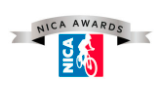NICA is pleased to recognize outstanding student-athletes, coaches and volunteers from across the United States with the 2020 NICA Awards. The NICA Awards are presented across eleven categories to twenty individuals who have been nominated by their peers as the most outstanding student-athletes, dedicated coaches and most supportive volunteers and sponsors that have contributed to the youth cycling movement during the past year.

“The 2020 NICA Award recipients exemplify our mission of building strong minds, bodies, character and communities through interscholastic cycling. Even during a year when activities were not normal due to the pandemic, our student-athletes, coaches, volunteers and community found new and innovative ways to embrace our values and continue to bring NICA’s mission to our community. Through their involvement and contributions to their leagues, teams and the broader NICA community, each recipient has made a unique and profound impact. We are thrilled to honor these individuals and highlight their contributions to our community. Congratulations to the 2020 NICA Award recipients!” says Steve Matous, NICA President.

Check out all of the 2020 NICA Awards Recipients HERE.

 table div table+table+table+table+table+table+table+table+table+table div table{width:100%;padding:0}table div table+table+table+table+table+table+table+table+table+table div table img{width:96.23%;padding:0;float:none}table div table+table+table+table+table+table+table+table+table+table div table td{width:100%;padding:0 1.88% 18px}/* styles */## Social Media Best Practices - Connecting with Your Community

 /* styles */ Social Media Questions? Reach out Emily McDonald - our Communications & Marketing Manger at emily@nationalmtb.org! NICA Virtual Communication Guidelines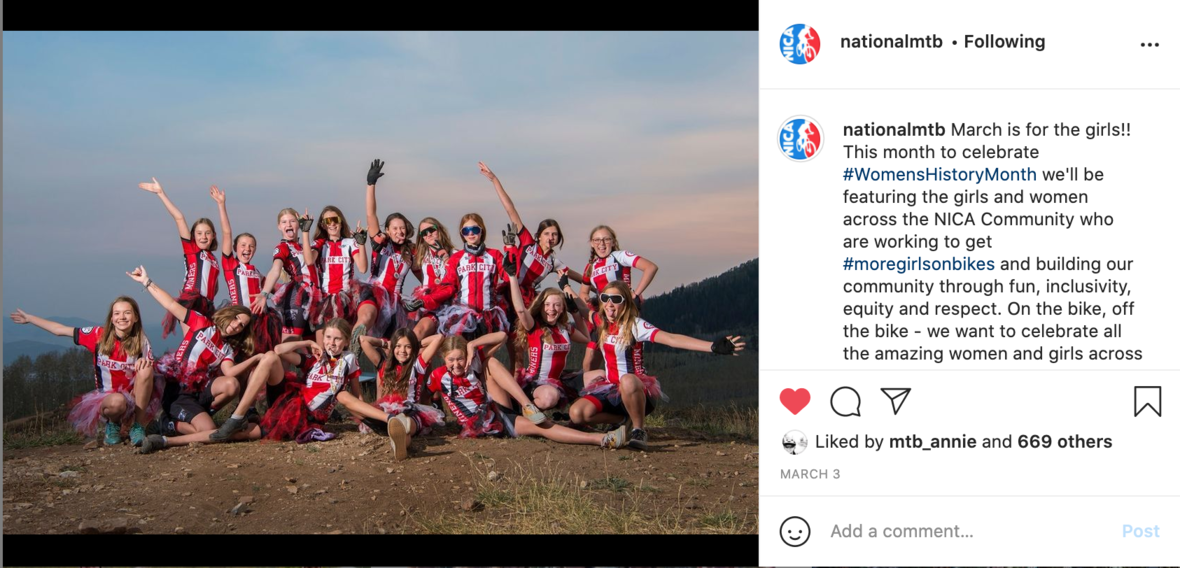/* styles */ Social Media is a powerful tool that you can use to: * Bolster awareness of your league / team * Engage with your community * Provide information and education * Thank and highlight sponsors * Connect with your community How do we connect with our community using social media? * Engage - comment on posts, share posts, respond to comments * Post - Show what you’re doing! * Repost - Repurposing existing posts. Great way to share content if you’re short on time or don’t have your own content. * Tag - Tag @nationalmtb and your league as well as sponsors. This expands your reach and lets us know what you’re doing! Popular Social Media Platforms * Facebook - Best for sharing longer posts, sharing links to articles or other websites, photo albums and videos * NICA now has the ability to fundraise via Facebook Nonprofits * Instagram - Popular with youth, primarily for images, video and “stories”. Connect to other users via tags and hashtags * Twitter - Town-hall style announcements, can share links and photos, character limited (240) * LinkedIn - Professional development and networking with like-minded individuals and the industry. Good place to share articles What to think about when posting: * BE Thoughtful in your posts * BE Creative * HAVE Fun! * PAUSE before you post * THINK if you’d want your loved ones to see the post * AVOID posting anything that wouldn’t be appropriate for NICA practices or events
 table div table+table+table+table+table+table+table+table+table+table+table+table+table+table+table div table{width:100%;padding:0}table div table+table+table+table+table+table+table+table+table+table+table+table+table+table+table div table img{width:96.23%;padding:0;float:none}table div table+table+table+table+table+table+table+table+table+table+table+table+table+table+table div table td{width:100%;padding:0 1.88% 18px}/* styles */## How to Find Your Coach Credentials

 /* styles */ We have been getting some questions about where to find and download coach license credentials lately. Here is an easy step-by-step guide to find your coach license. 1. Log into Pit Zone 2. Click on “Download License Document” 3. Save the PDF of your License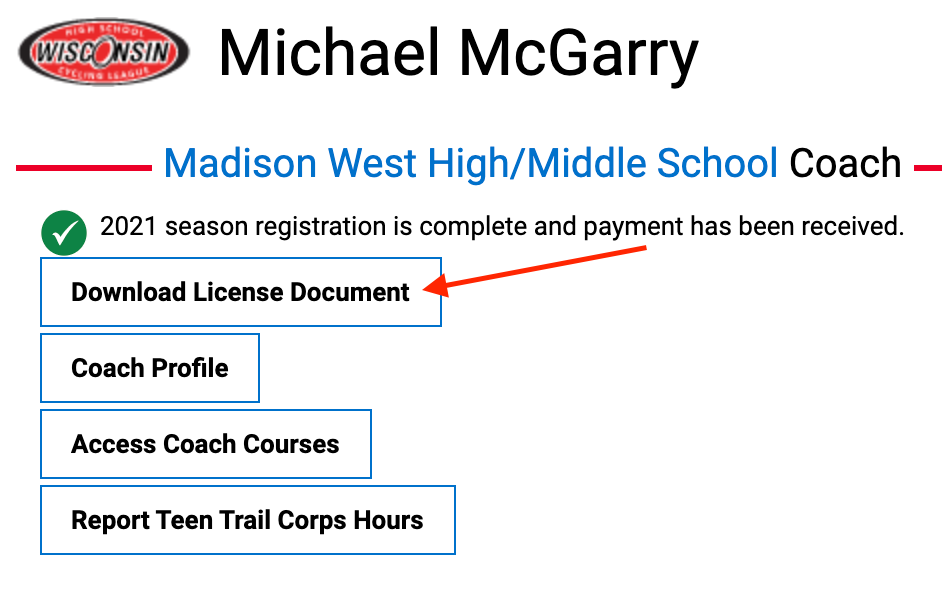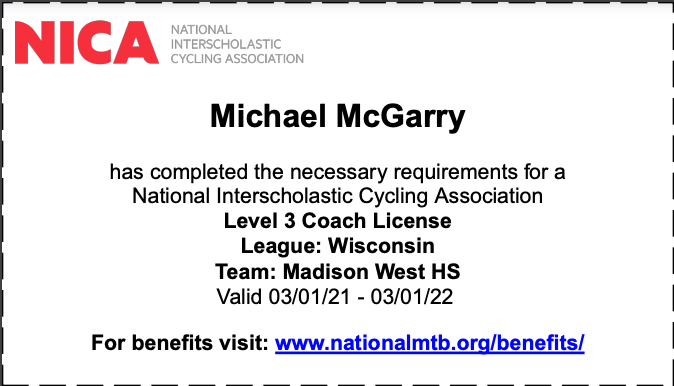table div table+table+table+table+table+table+table+table+table+table+table+table+table+table+table+table+table+table+table div table{width:100%;padding:0}table div table+table+table+table+table+table+table+table+table+table+table+table+table+table+table+table+table+table+table div table img{width:96.23%;padding:0;float:none}table div table+table+table+table+table+table+table+table+table+table+table+table+table+table+table+table+table+table+table div table td{width:100%;padding:0 1.88% 18px}/* styles */## Join the NICA Coach Licensing Team!

 /* styles */ NICA will be hiring a Coach Licensing Manager to help continue to train and support amazing coaches across the country. If you have a passion for teaching and supporting coaches to be their best apply here! Application will be accepted through March 29th.
 table div table+table+table+table+table+table+table+table+table+table+table+table+table+table+table+table+table+table+table+table+table+table div table{width:100%;padding:0}table div table+table+table+table+table+table+table+table+table+table+table+table+table+table+table+table+table+table+table+table+table+table div table img{width:96.23%;padding:0;float:none}table div table+table+table+table+table+table+table+table+table+table+table+table+table+table+table+table+table+table+table+table+table+table div table td{width:100%;padding:0 1.88% 18px}/* styles */# Trek/NICA Pathfinders Scholarship

Trek has partnered with NICA to grow diversity in the sport of cycling and provide better access for diverse and underrepresented youth.

The Trek/NICA Pathfinders Scholarship will provide selected student-athletes with a Trek Marlin mountain bike, helmet, shoes, accessories, and a kit, as well as a stipend for NICA League and entry fees and a full season of racing and events.

Applicants in Fall Leagues can apply for scholarships for up to 10 student-athletes. Applications are due April 15, 2021. A second round of applications for teams in Spring Leagues will open in August. Learn more and apply today HERE.

 table.module-24{width:75.47%;padding:0}table div table+table+table+table+table+table+table+table+table+table+table+table+table+table+table+table+table+table+table+table+table+table+table+table+table div table{width:75.47%;float:none;margin-left:auto;margin-right:auto;padding:0}table div table+table+table+table+table+table+table+table+table+table+table+table+table+table+table+table+table+table+table+table+table+table+table+table+table div table a{border:0 none;text-decoration:none}table div table+table+table+table+table+table+table+table+table+table+table+table+table+table+table+table+table+table+table+table+table+table+table+table+table div table img{width:100%!important;border:0 none;text-decoration:none}table div table+table+table+table+table+table+table+table+table+table+table+table+table+table+table+table+table+table+table+table+table+table+table+table+table div table td{width:100%;padding:0}/* styles */

# The Sparkle On Scholarship is open for applications!

Calling all graduating seniors headed to college in the fall that would love to continue racing! The Sparkle On Scholarship presented by Kate Courtney in partnership with Scott, Syncros, SRAM, and RockShox is open for applications through March 31st. Please make sure your graduating student-athletes know about this awesome opportunity.

The Scholarship will be awarded to four seniors from NICA leagues and consist of a monetary award of \$10,000 each, mentorship in the form of quarterly calls with Kate during their first year of college and career mentoring through SRAM. Those recipients without a bike sponsor and committing to race on Scott for the year will also receive a Scott mountain bike. The Sparkle On Scholarship is open to NICA student-athletes graduating high school who will attend college for the 2021-22 academic year and who are seeking to balance mountain bike racing with college studies. Find the application and FAQs here.

 table.module-26{width:75.47%;padding:0}table div table+table+table+table+table+table+table+table+table+table+table+table+table+table+table+table+table+table+table+table+table+table+table+table+table+table+table div table{width:75.47%;float:none;margin-left:auto;margin-right:auto;padding:0}table div table+table+table+table+table+table+table+table+table+table+table+table+table+table+table+table+table+table+table+table+table+table+table+table+table+table+table div table a{border:0 none;text-decoration:none}table div table+table+table+table+table+table+table+table+table+table+table+table+table+table+table+table+table+table+table+table+table+table+table+table+table+table+table div table img{width:100%!important;border:0 none;text-decoration:none}table div table+table+table+table+table+table+table+table+table+table+table+table+table+table+table+table+table+table+table+table+table+table+table+table+table+table+table div table td{width:100%;padding:0}/* styles */
 table div table+table+table+table+table+table+table+table+table+table+table+table+table+table+table+table+table+table+table+table+table+table+table+table+table+table+table+table div table{width:100%;padding:0}table div table+table+table+table+table+table+table+table+table+table+table+table+table+table+table+table+table+table+table+table+table+table+table+table+table+table+table+table div table img{width:96.23%;padding:0;float:none}table div table+table+table+table+table+table+table+table+table+table+table+table+table+table+table+table+table+table+table+table+table+table+table+table+table+table+table+table div table td{width:100%;padding:0 1.88% 18px}/* styles */## CampFire Chat - Weather Ready Mountain Biking

 table div table+table+table+table+table+table+table+table+table+table+table+table+table+table+table+table+table+table+table+table+table+table+table+table+table+table+table+table+table+table div table,table.module-29{width:37.74%;float:right;padding:0}table div table+table+table+table+table+table+table+table+table+table+table+table+table+table+table+table+table+table+table+table+table+table+table+table+table+table+table+table+table+table div table a{border:0 none;text-decoration:none}table div table+table+table+table+table+table+table+table+table+table+table+table+table+table+table+table+table+table+table+table+table+table+table+table+table+table+table+table+table+table div table img{width:100%!important;border:0 none;text-decoration:none}table div table+table+table+table+table+table+table+table+table+table+table+table+table+table+table+table+table+table+table+table+table+table+table+table+table+table+table+table+table+table div table td{width:100%;padding:0 0 20px 20px}/* styles */ NICA Campfire Chats are going to be a semi-regular series of moments for Student-Athletes from all leagues to come together in a virtual campfire setting. Some chats will be webinars covering topics throughout the world of mountain biking, while some chats will be activities or discussions that get student-athletes interacting with each other. Can you predict when your local trail will have “hero dirt” or be “underwater?” Make more confident weather planning decisions with guidance from AMB Weather Meteorologist, Kent McMullen. You’ll learn to best incorporate weather forecasts into your ride plans with a focus on heavy rain, rain accumulation threshold and lightning. Bring your own weather stories and questions! Register for the next Campfire Chat on March 24th at 8pm EST here
 table div table+table+table+table+table+table+table+table+table+table+table+table+table+table+table+table+table+table+table+table+table+table+table+table+table+table+table+table+table+table+table div table{width:100%;padding:0}table div table+table+table+table+table+table+table+table+table+table+table+table+table+table+table+table+table+table+table+table+table+table+table+table+table+table+table+table+table+table+table div table img{width:96.23%;padding:0;float:none}table div table+table+table+table+table+table+table+table+table+table+table+table+table+table+table+table+table+table+table+table+table+table+table+table+table+table+table+table+table+table+table div table td{width:100%;padding:0 1.88% 18px}/* styles */## Coach Licensing - Updated Courses and FAQs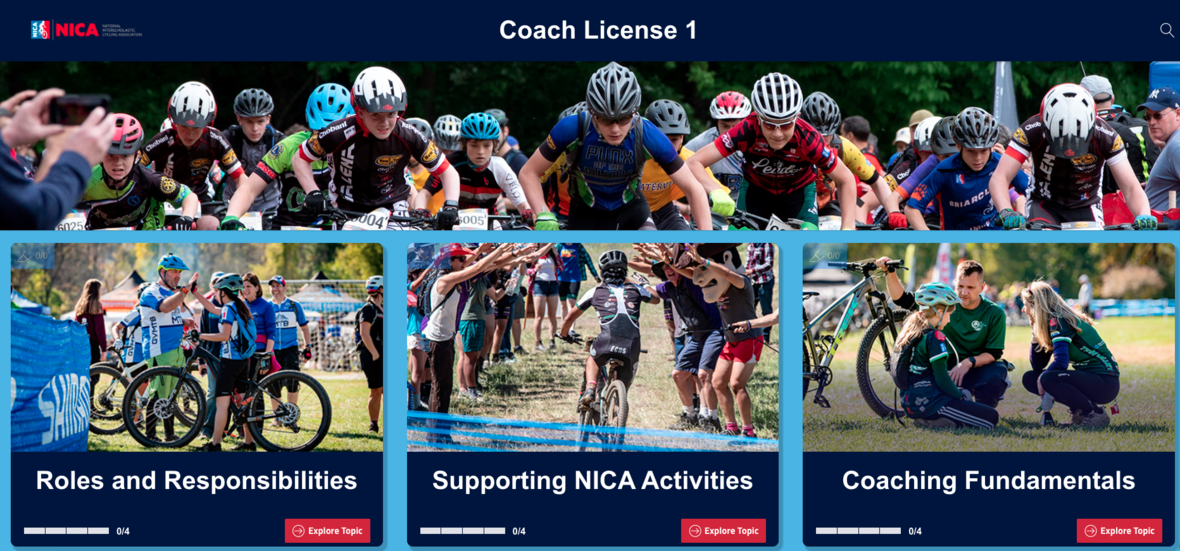Over the past year, the NICA Coach Licensing Team undertook a project to significantly enhance the substance and quality of the educational content contained in the Coach Licensing program. As of March 1st, 2021 when Pitzone opens for Fall Leagues, there will be new interactive e-learning courses for each license level.

VERY IMPORTANT: This does not apply to Spring Leagues until September 2021.

The way that you access the course will not change and we are not adding any new requirements. The content is more relevant to your work as coaches at all levels. The content is more engaging than watching a video. The learning is stickier so you will only need to take the Coach Licensing Level associated with your license every 2 years instead of annually.

Change can be intimidating and a little unnerving. We want to make this change as seamless as possible. Please review this FAQ about the update. Let us know if you have any remaining questions.

FAQ for Coach Licensing Revision

 table div table+table+table+table+table+table+table+table+table+table+table+table+table+table+table+table+table+table+table+table+table+table+table+table+table+table+table+table+table+table+table+table+table+table div table{width:100%;padding:0}table div table+table+table+table+table+table+table+table+table+table+table+table+table+table+table+table+table+table+table+table+table+table+table+table+table+table+table+table+table+table+table+table+table+table div table img{width:96.23%;padding:0;float:none}table div table+table+table+table+table+table+table+table+table+table+table+table+table+table+table+table+table+table+table+table+table+table+table+table+table+table+table+table+table+table+table+table+table+table div table td{width:100%;padding:0 1.88% 18px}/* styles */## Giddy Up for Good Challenge

 /* styles */ Join long time supporter, NICA advisory council member and world-class athlete Rebecca Rusch for the Giddy Up Challenge this Memorial Day Weekend, May 28-31. Pick an elevation climb from Quarter Everest to Full Everest. All NICA licensed coaches will receive 25% off of registration with the code NICAGUC21. A portion of the fundraising will benefit NICA.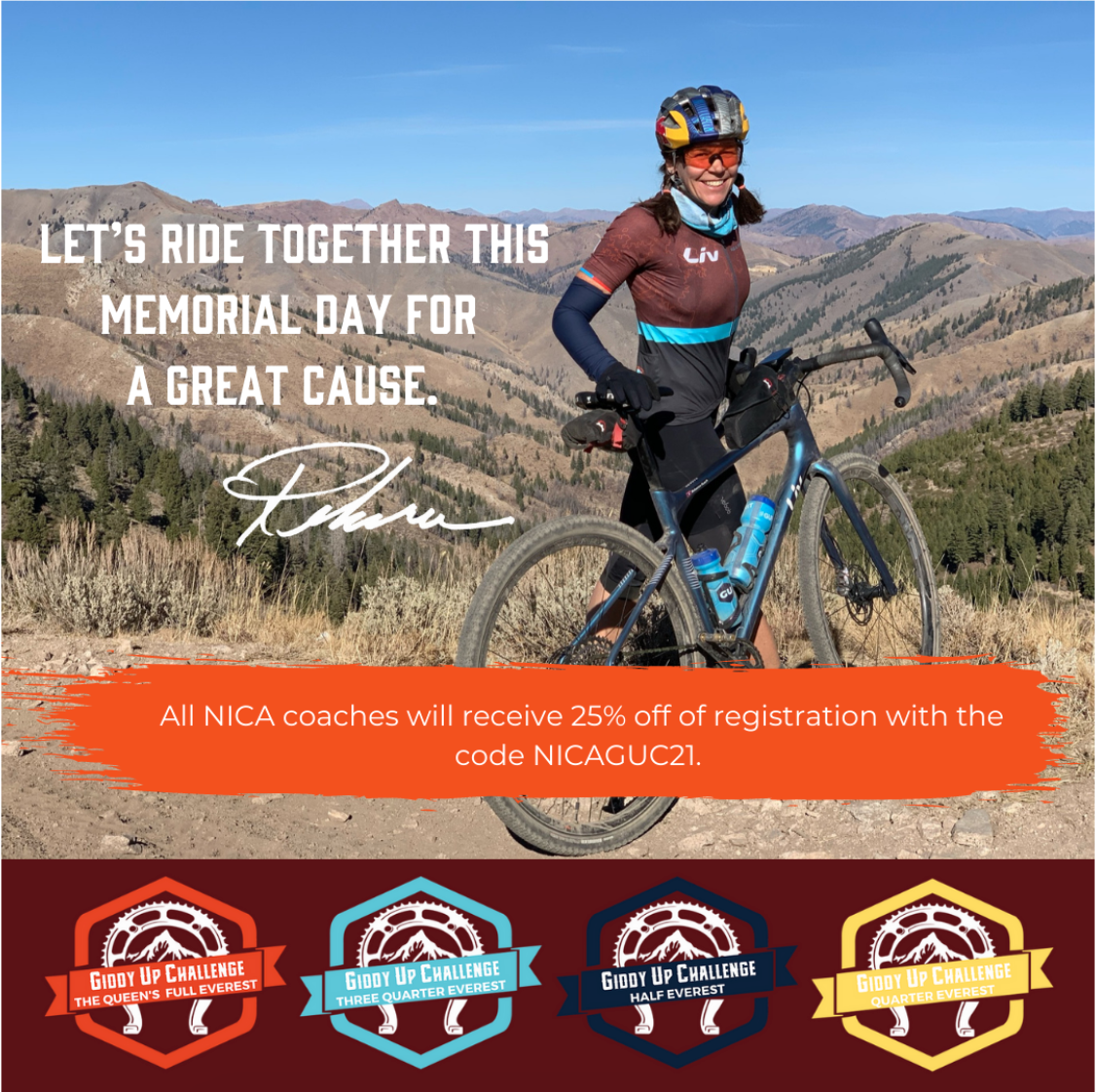Giddy Up for Good Challenge with Rebecca Rusch
 table div table+table+table+table+table+table+table+table+table+table+table+table+table+table+table+table+table+table+table+table+table+table+table+table+table+table+table+table+table+table+table+table+table+table+table+table+table+table div table{width:100%;padding:0}table div table+table+table+table+table+table+table+table+table+table+table+table+table+table+table+table+table+table+table+table+table+table+table+table+table+table+table+table+table+table+table+table+table+table+table+table+table+table div table img{width:96.23%;padding:0;float:none}table div table+table+table+table+table+table+table+table+table+table+table+table+table+table+table+table+table+table+table+table+table+table+table+table+table+table+table+table+table+table+table+table+table+table+table+table+table+table div table td{width:100%;padding:0 1.88% 18px}/* styles */## Strawberry meet Hibiscus! - New GU Energy Drink

 /* styles */ This new caffeine-free addition to the ROCTANE Energy Drink family is packed with carbs, electrolytes, and amino acids. The flavors of juicy strawberries and tart hibiscus come together in this crave-worthy drink that can fuel the toughest training sessions and races. This carbohydrate-intensive energy drink (250 calories, 320mg sodium, 1900mg BCAA) is designed to help athletes push their limits during ultra-distance and intense efforts. That's why it's no surprise ROCTANE Energy Drink is the secret weapon for a lot of podium finishes.
 table.module-40{width:38.49%;padding:0}table div table+table+table+table+table+table+table+table+table+table+table+table+table+table+table+table+table+table+table+table+table+table+table+table+table+table+table+table+table+table+table+table+table+table+table+table+table+table+table+table+table div table{width:38.49%;float:none;margin-left:auto;margin-right:auto;padding:0}table div table+table+table+table+table+table+table+table+table+table+table+table+table+table+table+table+table+table+table+table+table+table+table+table+table+table+table+table+table+table+table+table+table+table+table+table+table+table+table+table+table div table a{border:0 none;text-decoration:none}table div table+table+table+table+table+table+table+table+table+table+table+table+table+table+table+table+table+table+table+table+table+table+table+table+table+table+table+table+table+table+table+table+table+table+table+table+table+table+table+table+table div table img{width:100%!important;border:0 none;text-decoration:none}table div table+table+table+table+table+table+table+table+table+table+table+table+table+table+table+table+table+table+table+table+table+table+table+table+table+table+table+table+table+table+table+table+table+table+table+table+table+table+table+table+table div table td{width:100%;padding:0}/* styles */
 table div table+table+table+table+table+table+table+table+table+table+table+table+table+table+table+table+table+table+table+table+table+table+table+table+table+table+table+table+table+table+table+table+table+table+table+table+table+table+table+table+table+table div table{width:100%;padding:0}table div table+table+table+table+table+table+table+table+table+table+table+table+table+table+table+table+table+table+table+table+table+table+table+table+table+table+table+table+table+table+table+table+table+table+table+table+table+table+table+table+table+table div table img{width:96.23%;padding:0;float:none}table div table+table+table+table+table+table+table+table+table+table+table+table+table+table+table+table+table+table+table+table+table+table+table+table+table+table+table+table+table+table+table+table+table+table+table+table+table+table+table+table+table+table div table td{width:100%;padding:0 1.88% 18px}/* styles */## Pearl Izumi - Limited Time Coach Discount!

 /* styles */ For a limited time our friends at Pearl Izumi are offering coach benefits for all Level 3 coaches! To verify your license please submit your request on the Coach Benefit Form.table div table+table+table+table+table+table+table+table+table+table+table+table+table+table+table+table+table+table+table+table+table+table+table+table+table+table+table+table+table+table+table+table+table+table+table+table+table+table+table+table+table+table+table+table+table+table div table{width:100%;padding:0}table div table+table+table+table+table+table+table+table+table+table+table+table+table+table+table+table+table+table+table+table+table+table+table+table+table+table+table+table+table+table+table+table+table+table+table+table+table+table+table+table+table+table+table+table+table+table div table img{width:96.23%;padding:0;float:none}table div table+table+table+table+table+table+table+table+table+table+table+table+table+table+table+table+table+table+table+table+table+table+table+table+table+table+table+table+table+table+table+table+table+table+table+table+table+table+table+table+table+table+table+table+table+table div table td{width:100%;padding:0 1.88% 18px}/* styles */